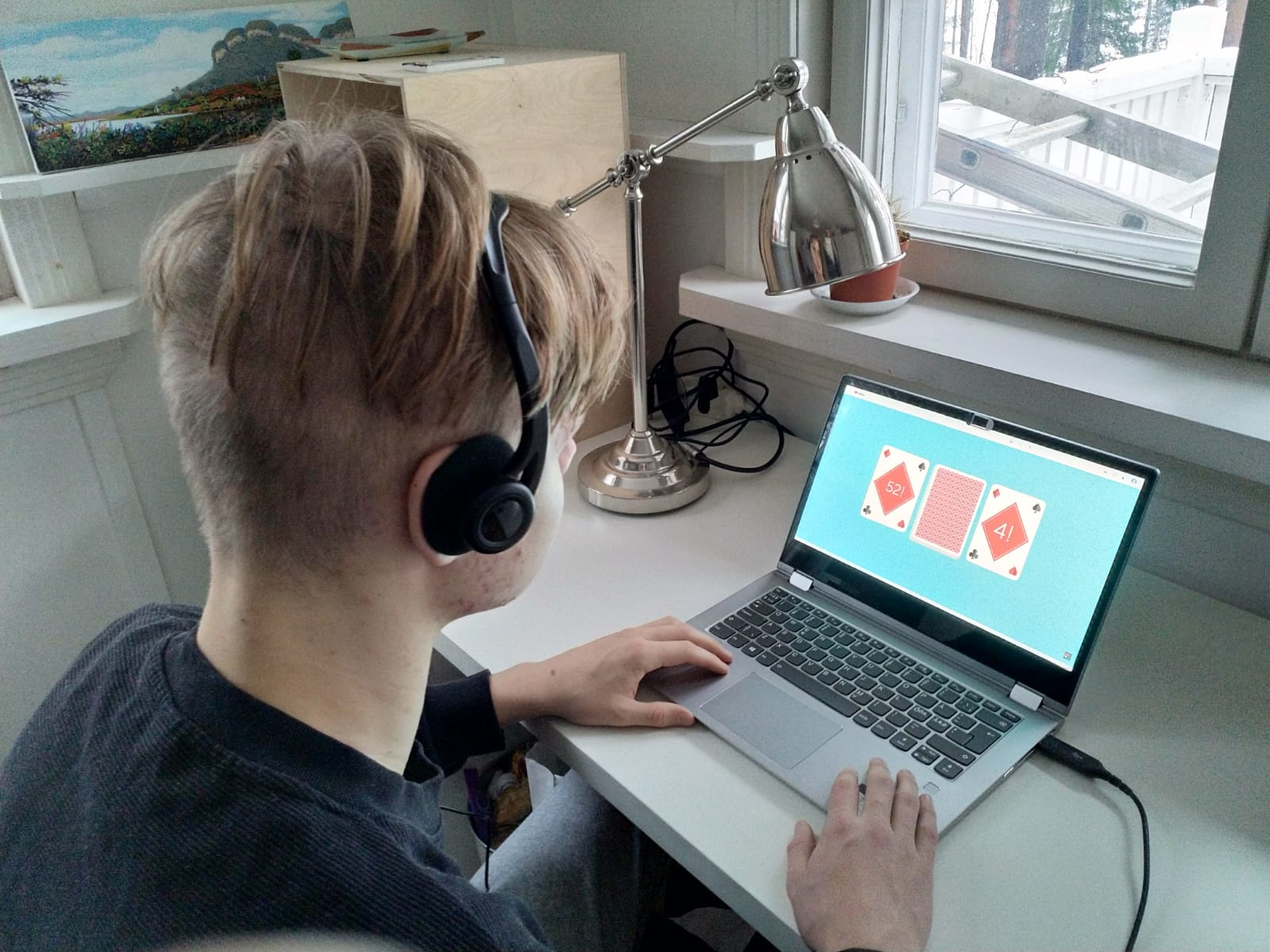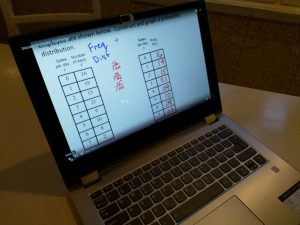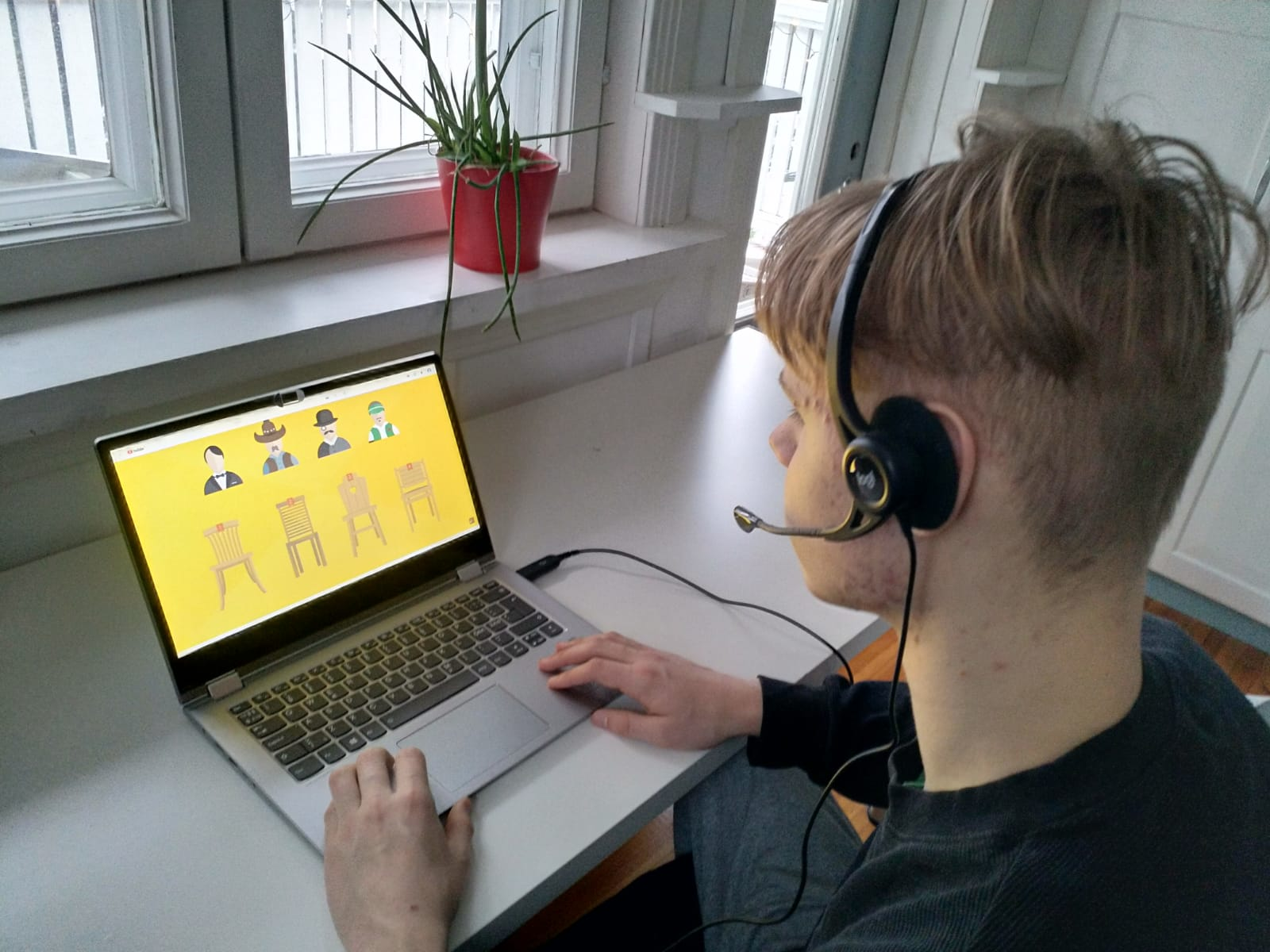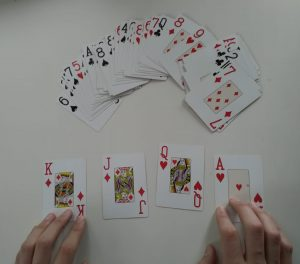Year 2020 has been a year of COVID 19 pandemic and distance teaching around the world. So these two lesson plans for high school math are designed for distance teaching. As all teachers in Finland, I had literally one day to prepare for distance teaching last spring. I had no previous experience about the distance teaching and was not familiar with all the applications needed for it. Well, that required some quick adaptation…I quickly decided that I would try to apply the same principles I regularly use in my classroom teaching. I’m a high school math teacher (in Finland high school is called general upper secondary school) and I teach students both in the IB program and those students who are studying according to the Finnish national curriculum for general upper secondary schools.

I personally think that the students’ own activity is the key to all learning. I try to leave as much time as possible in my lessons for the students’ own active participation (calculating, doing exercises, asking questions etc.). In my opinion that is how you really learn to understand and apply mathematics.

But I also think that in distance teaching it is wise to use different forms of teaching and demonstration material. For example, there are plenty of great math videos in Internet which illustrate and visualize complex phenomena in an understandable way. Why not use them to clarify new concepts to be learned?

In addition, I think allowing students to collaborate also in distance teaching helps them to really internalize the mathematical concepts. Peer learning is a great resource in developing the true grasp of mathematics. I personally think that keeping the teacher’s instruction short and clear works well in distance teaching, too.

So here are two distance teaching lesson plans for high school math where I have tried to apply these principles. We used Microsoft Teams application for conducting the lessons and digital learning platform Moodle for sharing the materials and submitting the exercises. High school lessons in Finland typically last 75 minutes. Enjoy!

#### 19th lesson: Discrete probability distribution

Thegoal of the lesson was for students to learn how to find the discrete probability distribution and the expected value. I used a sort of flipped learning method for this lesson. I asked students to watch a video about discrete probability distribution as a pre-assignment before the lesson. The video (https://www.youtube.com/watch?v=YZRKLYbxK3w) illustrates discrete probability distribution very well and students get a certain prior understanding of the phenomenon before the actual lesson.

In the beginning of the lesson, I used activating lecture method and gave students a short introduction (max 10 mins) to the topic covering following themes:

- What is a discrete probability distribution

- One example: If you throw a die, what are the probabilities for different results? Write down a table to show the results. What is the sample base?

Then I divided the students into pairs to work together online. I gave an exercise for the pairs to solve (10 mins): “Investigate the sum of two dice. Make a table of discrete probability distribution for the sum of the two dice. Send your solution to the teacher through the learning platform (Moodle).”

When students started submitting their answers to the learning platform, I quickly glanced through them and picked a couple of different solutions from different pairs to show for the whole class. We discussed the results with the students. One topic we talked about was what is the best way to find a sample base.

After this quick check-up (10 mins) and discussion, students continued to work in pairs. Next exercise I gave for the pairs: “Investigate the number of heads, when you throw three coins. What is the discrete probability distribution for the number of heads if you throw three dice? Another topic to ponder: What is the expected value in this case? (No correct answers needed; start discussing the concept of expected value). Send your results to the teacher through the learning platform (Moodle).” (10 mins for this exercise, too.)

Once again, we checked the results together. I showed different results from different pairs to the whole group (through the learning platform) and discussed the results with the students (15 mins). These were the topics we discussed particularly:

• What is the best way to find a sample base?
• How can you find the expected value? (Formula for the mean)
• Why don't we have a denominator in the expected value formula?

When students had grasped the idea of a sample base, expected value etc. through their own experiments and a joint teaching discussion, I gave them exercises from their book or digital material about the discrete probability distribution and expected value to do by themselves (20 mins for this). I encouraged them to ask if they had any problems and I was there to help them if needed. It was time for the students to apply this new learned information themselves.#### 20th lesson: Permutations

The goal of the lesson was that the students will understand the concept of permutations and are able to solve permutation problems.

I started with orientation (5 mins) by explaining the topic of today's lesson: permutation. I presented a question to think about - how many ways you can arrange a deck of cards? – and went through a definition of permutation (on a slide).

I had asked the students to have an ordinary 52 card deck available. Next I asked them to do the following exercise by themselves (20 mins):"Step 1: Take 2 different cards. In how many ways can you put them in a row? Take notes.

Step 2: Take 3 different cards. In how many ways can you put them in a row? Take notes.

Step 3: Take 4 different cards. In how many ways can you put them in a row? Take notes.

You can try to find the general rule behind the results."

At the end we had a joint discussion, and I asked several different students what results they got, but didn’t tell them the correct answers to the exercises yet.

Next, I showed the class a video (less than 10 mins) about permutations: How many ways can you arrange a deck of cards ( https://www.youtube.com/watch?v=uNS1QvDzCVw ).

After watching the video I went through the main points with a class using slides:

- What is the general rule of permutations?

- Giving one, slightly different example of permutations: If you have 6 different shirts, 5 different pair of pants and 8 different pair of shoes, how many different outfits (shirt + pants + shoes -combinations) can you form out of them?

After the instruction, it was time for the students to practice and apply the new information themselves. They did exercises from their math books / e-books by themselves (25 mins all together). I was available all the time online ready to answer questions, explain and offer support, if needed. Students could also work in pairs or in small groups if they wished so.

At the end of the lesson, we used about 5 minutes for reflection and had a short discussion together: What did we learn today? How was it? Do you have any further questions? I asked students to do the rest of the exercises they didn't manage to do during the lesson as a homework.

**

To be honest, I don’t think distance teaching mathematics is a piece of cake. There is almost inevitably less interaction with the students, which I think is a great loss. But using different pedagogical solutions helps to activate students and at least to some extent to overcome these challenges. LessonApp contains dozens of great teaching methods for distance teaching too and I highly recommend it! You can find these lesson plans of mine in LessonApp, too.I wish resilience and strength to all colleagues around the world! Let’s hope that year 2021 is much better for all of us!

All the best,

Tero Taubert